PROJECT ENGG » AIRCRAFTS » COCKPIT SEAT K12-7550-0

## The geometry of the design pilot's seat K12-7550-0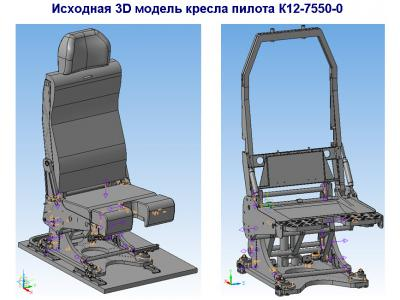The geometry of the design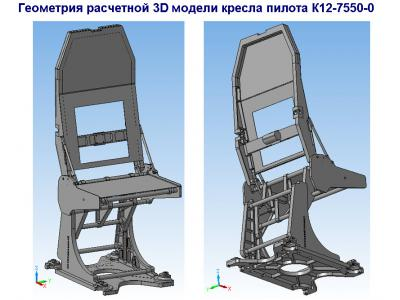The geometry of the design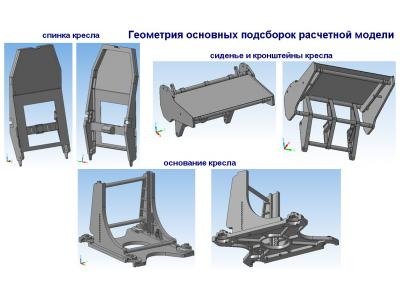The geometry of the design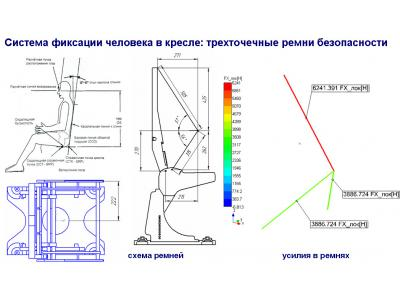The load on the model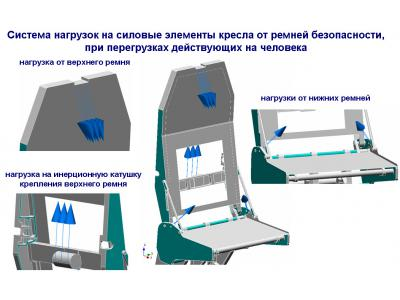The load on the model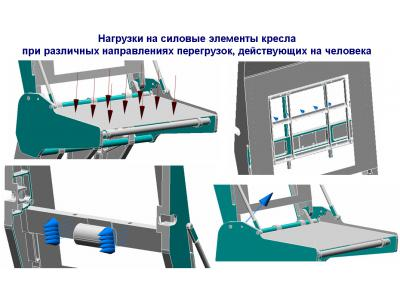The load on the model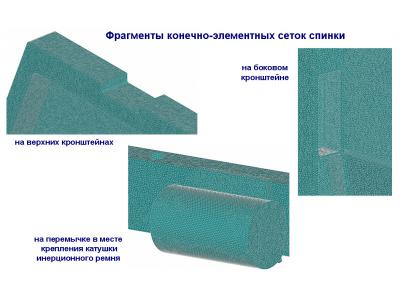The finite-element meshThe finite-element mesh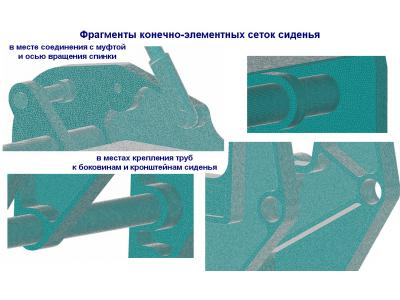The finite-element mesh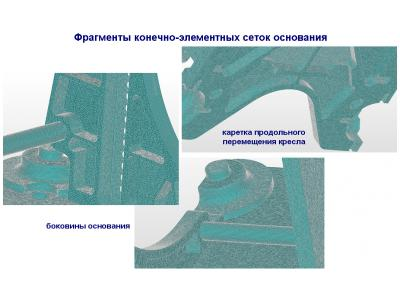The finite-element mesh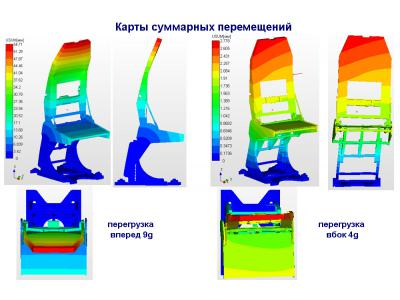The calculation results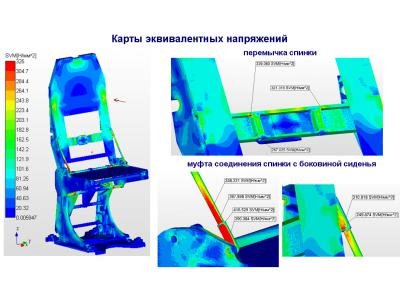The calculation results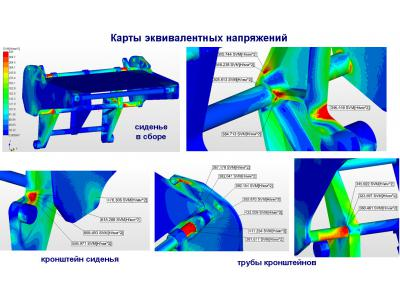The calculation results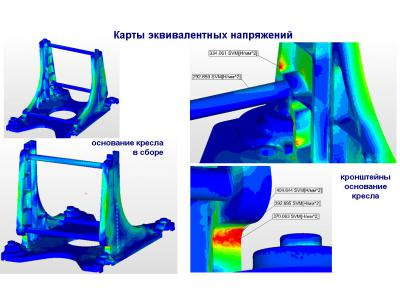The calculation results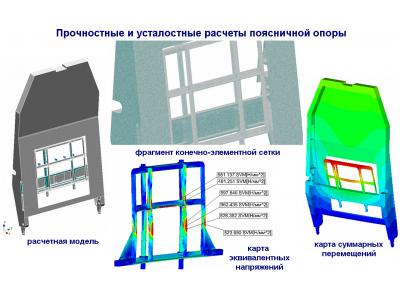The calculation results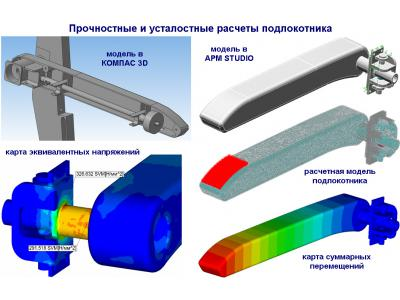The calculation results

info@paviathintegratedsolution.com www.paviathintegratedsolution.com